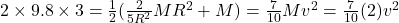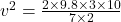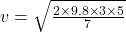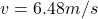## A solid ball of mass M = 2.0 kg and radius R = 0.25 m starts from rest at a height h = 3.0 m above the bottom of the path. It rolls without

Question

A solid ball of mass M = 2.0 kg and radius R = 0.25 m starts from rest at a height h = 3.0 m above the bottom of the path. It rolls without slipping down the left side of the path. The right side of the path is frictionless. Moment of inertia of a hollow sphere is � = ! ! ��!. v What is the linear speed

in progress 0
2 months 2021-07-22T12:30:10+00:00 1 Answers 3 views 0

6.48 m/s

Explanation:

We are given that

Mass,M=2 kg

Height,h=3 m

Moment of inertia of solid sphere=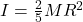We have to find the linear speed.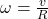By law of conservation of energy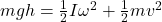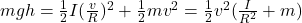Where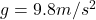Substitute the values